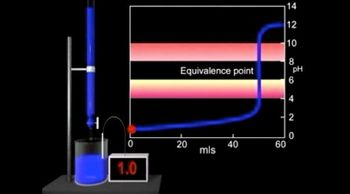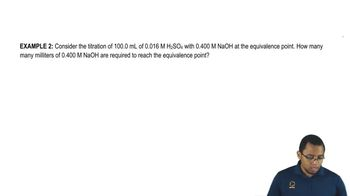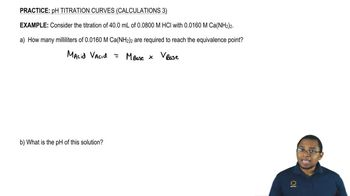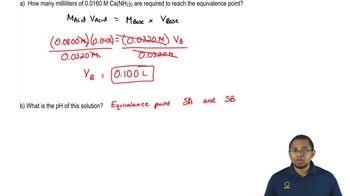Start typing, then use the up and down arrows to select an option from the list.Jules Bruno
182views
1
5
Hey, guys, In this new video, we're gonna continue with our discussion of Ph tight Trish in curves when looking at indicators. So in this first example, it says the acid form of an indicator And what we should realize here is that an indicator is really just a weak acid. So an indicator has an acidic h on it. So the acid form is gonna be h A. And it's an ion form Means it's removed. It's h so not a minus. So the acid form of an indicator is red and it's an ion form. Its conjugate base form is blue. The value for this indicator is 10 to the negative nine. What will be the approximate ph range over which this indicator changes color. Now, what you should realize here is that in order to figure out the pH range oven indicator, all you have to remember is this The pH range of an indicator equals PK plus or minus one. So we have the K. So how do we find a peek? A. If we have k, just take the negative log of it. So we're gonna say p k equals negative log of K a so equals negative. Log off 10 to the negative nine. So the P K equals nine. And we just said that the PH range for an indicator is PK a plus or minus one. So the ph range is nine plus or minus one, which means nine minus one is eight to nine, plus one is 10. So the range would be between eight and 10. And that's what we have to remember Now. I'm gonna give you guys a hint on the next question, so just understand it like this. We're going to say we have a peek A that is equal to nine. Well, we should realize here, if your P H is equal to nine, we have pH and PK equally each other. And what did we say about that? We said that when pH equals P k, you're at the half equivalents points, so you have equal amounts off your acid form and your base form. So at this moment, h A equals a minus. And we're gonna say here at P K nine, that means that the Ph has to be at least nine for us to start to remove the acidic H off of our indicator. So what color would our indicator be below Ph of nine? What color would our indicator be above ah ph of nine. This will help us understand the next practice question. So remember this pattern attempt to answer this question on your own and then don't worry, Come back. Look at the next following video. You'll see an example of me explaining how to approach this problem and what the correct answer is. Good luck, guys.1:259:0004:1301:3906:4303:0606:2002:2701:27